## Example Questions

### Example Question #1 : How To Find Out If A Number Is Prime

A prime number is divisible by:

Possible Answers:

It depends on the prime number.

ItselfTwo answers are correct.Correct answer:

Two answers are correct.

Explanation:

The definition of a prime number is a number that is divisible by only one and itself. A prime number can't be divided by zero, because numbers divided by zero are undefined. The smallest prime number is 2, which is also the only even prime.

### Example Question #16 : Integers

What is half of the third smallest prime number multiplied by the smallest two digit prime number?

Possible Answers:

This number does not exist.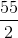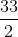Correct answer:Explanation:

The third smallest prime number is 5. (Don't forget that 2 is a prime number, but 1 is not!)

The smallest two digit prime number is 11.

Now we can evaluate the entire expression: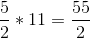### Example Question #17 : Integers

a, b and c are integers, and a and b are not equivalent.

If ax + bx = c, where c is a prime integer, and a and b are positive integers which of the following is a possible value of x?

Possible Answers:

2

4

1

None of the other answers

3

Correct answer:

1

Explanation:

This question tests basic number properties. Prime numbers are numbers which are divisible only by one and themselves. Answer options '2' and '4' are automatically out, because they will always produce even products with a and b, and the sum of two even products is always even. Since no even number greater than 2 is prime, 2 and 4 cannot be answer options. 3 is tempting, until you remember that the sum of any two multiples of 3 is itself divisible by 3, thereby negating any possible answer for c except 3, which is impossible. There are, however, several possible combinations that work with x = 1. For instance, a = 8 and b = 9 means that 8(1) + 9(1) = 17, which is prime. You only need to find one example to demonstrate that an option works. This eliminates the "None of the other answers" option as well.

### Example Question #81 : Arithmetic

Which statement is false about prime numbers?

Possible Answers:

There are no negative primes.

Every positive integer except for 1 is a prime or a product of primes.

None are false.

Every integer greater than 1 has a unique prime factorization.

All are false.

Correct answer:

None are false.

Explanation:

All of these statements are true. Let's go through them.

1. There are no negative prime numbers. This appears as if it might be false, but in fact, the prime numbers are defined as whole numbers greater than one that are divisible by only one and itself.

2. Every number except 0 and 1 is a prime number or product of primes. This is also true. Let's look at the factorization of a number that isn't prime. For example, 6 = 2 * 3, which is a product of primes.  12 = 2 * 2 * 3, which is also a product of primes.

3. Every number has a unique prime factorization. We just saw that every number is either prime, or a product of primes. Therefore each number must have a unique prime factorization. Just as above, 6 is the product of two primes, 2 and 3. No other number can be made by mulitplying 2 * 3. The same is true for 12. When we multiply 2 * 2 * 3, the only number we will ever get is 12.

### Example Question #1 : Other Factors / Multiples

Column A

5!/3!

Column B

6!/4!

Possible Answers:

The quantity in Column A is greater.

The quantity in Column B is greater.

The two quantities are equal.

The relationship cannot be determined from the information given.

Correct answer:

The quantity in Column B is greater.

Explanation:

This is a basic factorial question. A factorial is equal to the number times every positive whole number smaller than itself. In Column A, the numerator is 5 * 4 * 3 * 2 * 1 while the denominator is 3 * 2 * 1.

As you can see, the 3 * 2 * 1 can be cancelled out from both the numerator and denominator, leaving only 5 * 4.

The value for Column A is 5 * 4 = 20.

In Column B, the numerator is 6 * 5 * 4 * 3 * 2 * 1 while the denominator is 4 * 3 * 2 * 1. After simplifying, Column B gives a value of 6 * 5, or 30.

Thus, Column B is greater than Column A.

### Example Question #20 : Integers

Which of the following integers are factors of both 24 and 42?

Possible Answers:
12
8
7
3
5
Correct answer: 3
Explanation:

3 is the only answer that is a factor of both 24 and 42. 42/3 = 14 and 24/3 = 8.  The other answers are either a factor of 24 OR 42 or neither, but not both.

### Example Question #81 : Arithmetic

721(413) + 211(721) is equal to which of the following?

Possible Answers:

413(721 + 211)

(721 + 211)(413 + 721)

(721 + 413)(211 +721)

721(413 + 211)

211(413 + 721)

Correct answer:

721(413 + 211)

Explanation:

The answer is 721(413 + 211) because we can pull out a common factor, or 721, from both sides of the equation.

### Example Question #81 : Arithmetic

n is a positive integer .  p = 4 * 6 * 11 * n

Quantity A: The remainder when p is divided by 5

Quantity B: The remainder when p is divided by 33

Possible Answers:

The two quantities are equal.

Quantity A is greater.

Quantity B is greater.

The relationship cannot be determined from the information given.

Correct answer:

The relationship cannot be determined from the information given.

Explanation:

Let's consider Quantity B first.  What will the remainder be when p is divided by 33?

4, 6 and 11 are factors of p which means that 2 * 2 * 2 * 3 * 11 * n will equal p.  We can group the 3 and 11 to see that 33 will always be a factor of p and will have no remainder. Thus Quantity B will always equal 0 no matter the value of n.

Now consider Quantity A. Let's consider first the values for p when n equals 1 through 5. When n = 1, p = 264, and the remainder is 4/5 or 0.8.

n = 2, p = 528, and the remainder is 3/5 or 0.6.

n = 3, p = 792, and the remainder is 2/5 or 0.4.

n = 4, p = 1056, and the remainder is 1/5 or 0.2.

n = 5, p = 1320, and the remainder is 0 (because when n = 5, 5 becomes a factor of p and thus there is no remainder.

Because Quantity A can be equal to or greater than B, there is not enough information given to determine the relationship.

### Example Question #81 : Arithmetic

Quantitative Comparison

Quantity A: number of 2's in the prime factorization of 32

Quantity B: number of 2's in the prime factorization of 60

Possible Answers:

Quantity B is greater.

Quantity A is greater.

The relationship cannot be determined from the information given.

The two quantities are equal.

Correct answer:

Quantity A is greater.

Explanation:

32 = 2 * 16 = 2 * 4 * 4 = 2 * 2 * 2 * 2 * 2 = 25, so Quantity A = 5.

60 = 2 * 30 = 2 * 6 * 5 = 2 * 2 * 3 * 5 = 22 * 3 * 5, so Quantity B = 2.

Quantity A is greater.  Even though 60 is a larger number than 32, 32 has more 2's in its prime factorization.

### Example Question #1 : How To Factor A Number

Ifis an integer and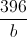is an integer, which of the following could be the value of?

Possible Answers:Correct answer:Explanation:

Because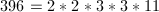, the answer choice that has a factorization set that cancels out completely with 396 will produce an integer. Only 18 fits this qualification, since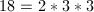.

### All GRE Math Resources